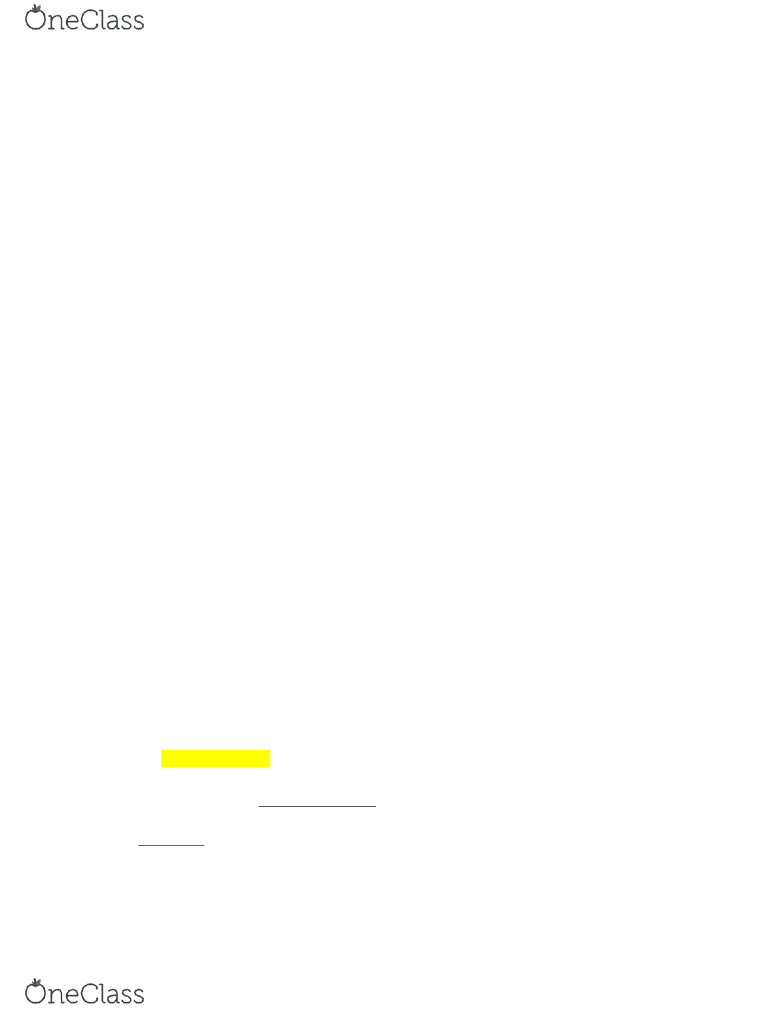Textbook Notes (280,000)
US (110,000)
UB (400)
CHE (10)
CHE 101 (10)
Chapter 10

# CHE 101 Chapter Notes - Chapter 10: Van Der Waals Equation, Molar Mass, Gas Laws

This preview shows pages 1-2. to view the full 8 pages of the document.Chapter 10 - Gases
Jim Atwood
A. Definitions
1. Gas - Molecules of a ga are not held together, expand to fill the space available
and can be compressed
2. Volume is the space occupied
1 Liter = 1 decimeter
1 cubic centimeter (cc) = 1 mL
1 L = 1000 mL = 1000 cc
3. Temperature Scales
° = ° − 
K = C° + 273.15
        −
4. Pressure
Measured as force acting on an area
P = (F / A)
F = force
A = area
In pounds / in2
  −   2)) / m2 which is a Pascal (Pa) *don’t need to
know
We primarily use relative measurements of pressures, since we are always
surrounded by gases
A barometer at sea level will measure 760 mm of Hg - defined as 1 atm
1 atm = 760 mm Hg
1 mm Hg = 1 Tor
STP (standard temperature and pressure) 1 atm (760 mm Hg) and 0°C
(273 K)
Example: Convert 0.605 atm into pressure in Torr.
(0.605 atm)(760 Torr / 1 atm) = 460 Torr
B. Gas Laws
1.   V  − ’ ,  
As volume decreases, pressure increases
1 L at 1 atm
0.5 L at 2 atm
find more resources at oneclass.com
find more resources at oneclass.com

Only pages 1-2 are available for preview. Some parts have been intentionally blurred.Two Expressions
V ∝ 
PV = constant
2. V    − ’ 
V ∝ 
T is in Kelvin
10°C → 20°C ***no change
Above statement is equivalent to 283 K → 293 K
At constant pressure the volume of a given quantity of gas is proportional
to absolute temperature.
3.   V  − ’ 
Gay-Lussac’s combining volumes
H2 + Cl2 → 2HCl
1 volume + 1 volume → 2 volumes
Equal volumes at the same pressure and temperature contain equal
numbers of molecules.
V = k N
N is number of molecules
V α N
V α n
Molar Volume
1 mole O2 −       
1 mole H2 −       
at STP (273.15 K and 1 atm) 1 mole of any gas occupies 22.4 L
C. Ideal Gas Equations
V α 1 / P
V α T
V α (nT) / P
V α n
V = (nRT) / P
R is the gas constant
The Ideal Gas Equation, which has all the variables that describes a gas
Example 1: Calculate the value of R
PV = nRT
R = (PV) / (nT)
R = ((1)(22.4)) / ((1)(273))
find more resources at oneclass.com
find more resources at oneclass.com

Unlock to view full version

Only pages 1-2 are available for preview. Some parts have been intentionally blurred.P = 1.00 atm
V = 22.4 L
n = 1.00 mol
T = 0°C + 273 = 273 K
R = 0.821 ((L)(atm)) / ((mol)(K))
Example 2: Calculate the pressure exerted by 18 g of steam confined to 18 L at 100°C.
PV = nRT
P = (nRT) / V
P = ((1)(0.0821)(373)) / (18)
n = (18 g H2O) / (1 mol H2O / 18 g H2O) = 1 mol
R = 0.0821
T = 100°C + 273 = 373 K
V = 18 L
P = 1.7 atm
Example 3: What volume would be required to contain 3.00 moles of O2 at 100°C and
2.00 atm pressure?
PV = nRT
V = (nRT) / P
V = ((3.00)(0.821)(373)) / (2.00)
n = 3.00 mol
R = 0.0821
T = 100°C + 273 = 373 K
P = 2.00 atm
V = 45.9 L
You should be able to solve any problem given three of the four variables.
1. Relation to other gas laws:
a. For a given quantity of gas at constant temperature
Boyle’s Law
PV = nRT = constant
P1V1 = P2V2
b. For a given quantity of gas at constant pressure
Charles’ Law
PV = nRT
V / T = nR / P = constant
V1 / T1 = V2 / T2
c. For a given quantity of gas
PV = nRT
(PV) / (T) = nR = constant
find more resources at oneclass.com
find more resources at oneclass.com